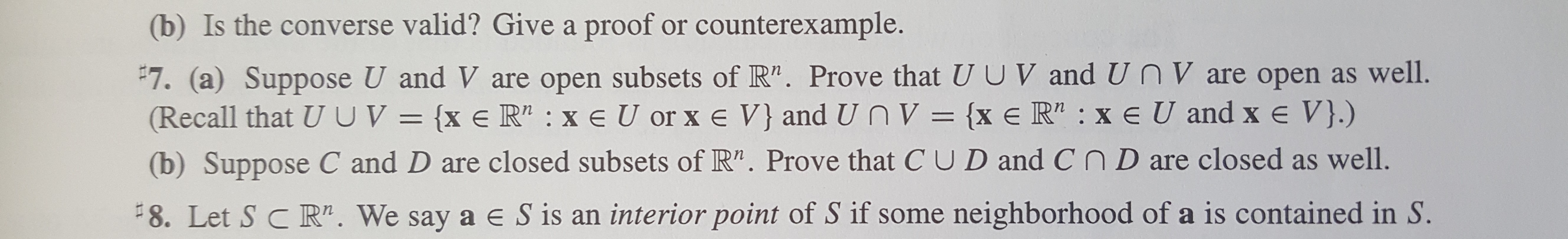# (b) Is the converse valid? Give a proof or counterexample.#7. (a) Suppose U and V are open subsets of R". Prove that U U V and UnV are open as well.(Recall that UUV= {x e R2 xe U or x e V} and Un V = {x e R" : x e U and x e V}.)(b) Suppose C and D are closed subsets of R". Prove that C UD and CnD are closed as well.8. Let S C R". We say a e S is an interior point of S if some neighborhood of a is contained in S.

Question
39 views

I need help for number 7. Thanks!help_outlineImage Transcriptionclose(b) Is the converse valid? Give a proof or counterexample. #7. (a) Suppose U and V are open subsets of R". Prove that U U V and UnV are open as well. (Recall that UUV= {x e R2 xe U or x e V} and Un V = {x e R" : x e U and x e V}.) (b) Suppose C and D are closed subsets of R". Prove that C UD and CnD are closed as well. 8. Let S C R". We say a e S is an interior point of S if some neighborhood of a is contained in S. fullscreen
check_circle

star
star
star
star
star
1 Rating
Step 1

(a)

Let

Step 2

Then by definition, either x belongs to U or x belongs to V.

Assume that x belongs to U,

Since, U is open, there is some r > 0 so that the open ball,

Step 3

Thus, union of two open subsets...

### Want to see the full answer?

See Solution

#### Want to see this answer and more?

Solutions are written by subject experts who are available 24/7. Questions are typically answered within 1 hour.*

See Solution
*Response times may vary by subject and question.
Tagged in

### Math